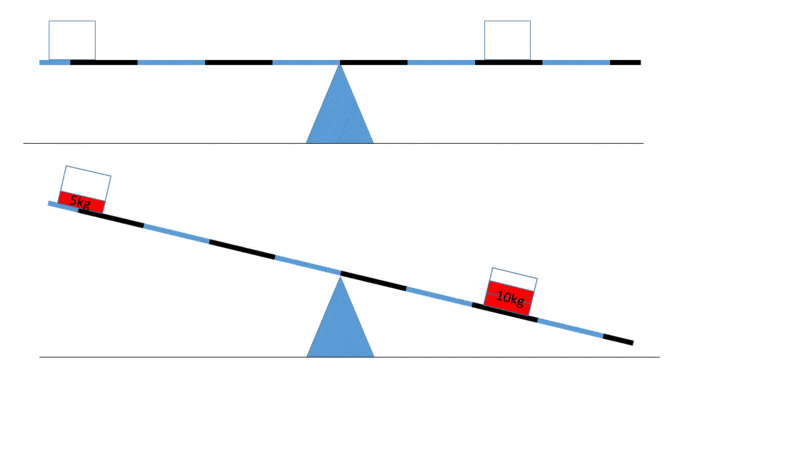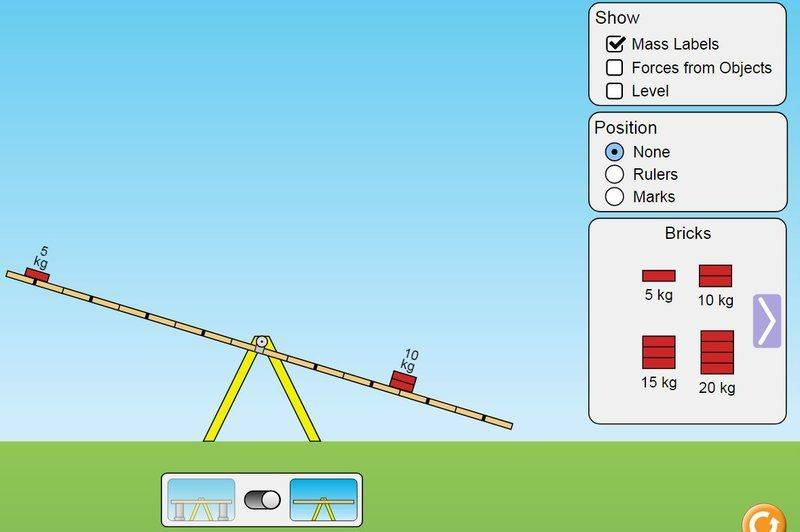# Irreversible machines

• B
Dimani4
Hi all,

I still have "a many times discussed" problem to understand Feymann's issue about irreversible/reversible machines example.

Here is the part from the original text.
We imagine that there are two classes of machines, those that are not reversible, which includes all real machines, and those that are reversible, which of course are actually not attainable no matter how careful we may be in our design of bearings, levers, etc. We suppose, however, that there is such a thing—a reversible machine—which lowers one unit of weight (a pound or any other unit) by one unit of distance, and at the same time lifts a three-unit weight. Call this reversible machine, Machine A. Suppose this particular reversible machine lifts the three-unit weight a distance X. Then suppose we have another machine, Machine B, which is not necessarily reversible, which also lowers a unit weight a unit distance, but which lifts three units a distance Y. We can now prove that Y is not higher than X; that is, it is impossible to build a machine that will lift a weight any higher than it will be lifted by a reversible machine. Let us see why. Let us suppose that Y were higher than X. We take a one-unit weight and lower it one unit height with Machine B, and that lifts the three-unit weight up a distance Y. Then we could lower the weight from Y to X, obtaining free power, and use the reversible Machine A, running backwards, to lower the three-unit weight a distance X and lift the one-unit weight by one unit height. This will put the one-unit weight back where it was before, and leave both machines ready to be used again! We would therefore have perpetual motion if Y were higher than X, which we assumed was impossible. With those assumptions, we thus deduce that Y is not higher than X, so that of all machines that can be designed, the reversible machine is the best.

Here is my questions:

1. Here Feymann talks about the situation when you are not applying any force to rise or lower the weight. Your system is closed (isolated) for any applying force, you only supply the weights for the system. Let's talk about irreversible machine like Feymann says
those that are not reversible, which includes all real machines,
Here in the case of irreversible machine we don't supply any energy to rise the weight. But if we do not supply the energy and the machine returns to the initial state and in the end the weight is raised we have the perpetual movement.

Take a look at the picture of the real irreversible machine:The first picture is perfectly balanced condition with baskets for weights. Let's neglect the weights of baskets and weight of lever and friction of fulcrum. Now, we put the weights into the baskets and then we have 5kg is lifted and 10 kg is lowered. Now we remove the weights and the scales back to the balanced condition. So we didnt apply any energy to lift the weight and the system back to the initial condition and in the end we succeeded to arise the weight that can do something else (as Feynman says)
we can use that lifted weight to run something else.
. So we have perpetual motion (according Feynman definition) here? If we do it again we will lift another 5kg and again 5kg...
If, when we have lifted and lowered a lot of weights and restored the machine to the original condition, we find that the net result is to have lifted a weight, then we have a perpetual motion machine because we can use that lifted weight to run something else.

#### Attachments

rumborak
Hi all,

Take a look at the picture of the real irreversible machine:

[...] Let's neglect the weights of baskets and weight of lever and friction of fulcrum.

I think with that last statement you switched back from irreversible to reversible machine.

Dimani4
I think with that last statement you switched back from irreversible to reversible machine.
Hi,
Why do you think so? I neglect it to return this machine to initial horizontal state. But anyway if you place the weights of 5kg and 10kg in the places as depicted in the figure it will always lift the 5kg and lower the 10kg-irreversible behavior.

rumborak
Well, the inability of returning it to the original state without friction losses is the very point of Feynman; it is the difference between a real machine (irreversible) and an idealized one (reversible).
In this universe, you can only build real machines.

•Dimani4
Dimani4
I think I know where I was wrong. Forget all the losses. I forgot about the 10kg. After the action (Figure 1 case2) 10kg is below the balanced state. To back it to the balanced state (horizontal state) we should apply work against the gravitational energy to put it back. There is no such a problem with 5kg. 5kg is above the balanced state (horizontal state). You see, to back this machine to the initial state we should do work to return 10kg to the balanced state, i.e. we should interfere in the isolated system (to let Bruce bring his blocks). But it isn't the case of perpetual mobile system. Perpetual mobile system should be isolated (closed) from interference from outside and give free energy forever. So this system can be work as perpetual mobile. But in the case of Feynman example to do perpetual mobile we should use reversible machine to get back the weights to the balanced state. But another condition for the perpetual motion we need to lift our weight with irreversible machine higher than reversible machine can in order to get free energy.

Last edited:
rumborak
I can't quite tell whether you drew the correct conclusion or not, but the bottom line of all of this is:

- Energy is conserved at all times
- All real machines incur irreversible losses

•Dimani4
Dimani4
You are absolutely right. Finally I understood the point I couldn't understand. why we can't build perpetual mobile with irreversible machine. Now I understand. We need to do work to get back 10kg to the initial state. This is it. I understand why reversible machines you can't build. Because in order to incline the lever to one of the sides you have to do some work "even infinitesimal", that's why Feymann called it "almost reversible" (even if you are neglecting friction). But if you are not neglecting friction you should do a bit more work to incline the lever to one of the sides.

Mentor
Your system is closed (isolated) for any applying force, you only supply the weights for the system. Let's talk about irreversible machine like Feymann says Here in the case of irreversible machine we don't supply any energy to rise the weight. But if we do not supply the energy and the machine returns to the initial state and in the end the weight is raised we have the perpetual movement.

Take a look at the picture of the real irreversible machine:

View attachment 207831

•